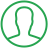# How To Find Data Types In Python

Last Updated : Jul 1, 2023In this article we will show you the solution of how to find data types in python, data types are one of the building blocks of any programming language. No programming language can exist without the data type and variables.

It's also important to know the data types defined in a programming language, to work in that language, Python is no exception for the same.

## Step By Step Guide On How To Find Data Types In Python :-

There are several ways to find the data type of a variable in Python, one of which is to use the built-in type() function.

The built-in type() function takes an argument and returns the data type of the object. This is the most followed approach to finding the data type of a variable in Python.

Yet, there is another approach, where we can use another built-in function, which is the isinstance() function.

`Syntax : isinstance(object, classtype)`

The isinstance() function, takes two arguments, the first is the object i.e., the variable name, and the second one is the classtype that can be a class, a type, or a tuple of types and classes.

As everything in Python is an object, and there are predefined classes in the language, the built-in isinstance() function checks if the given variable or the passed argument is an instance of the specific class type.

The isinstance() function returns True if the given object is of the given type, and returns False if the given object is not of the given type.

For example, if we passed a variable that has a type of int, but in place of the classtype we passed the type as string, then the output of the function will be False.

## Step By Step Guide On How To Find Data Types In Python :-

```pi = 3.14
print("pi is a float value:", isinstance(pi, float))
greet = "Hello World!"
print("greet is an integer value:", isinstance(greet, int))

#Code Output
pi is a float value: True
greet is an integer value: False```
1. As an initial step, we declare the variable pi and assign the value to it.
2. In the second step, we used the print() function to get the output on the console, with the isinstance() function inside it.
3. In the isinstance() function, we gave two arguments, first is the name of the variable i.e., pi, and second is we have given a data type of the variable as a float.
4. In the output, we can see that the isinstance() function has given the output as 'True'.
5. It means, that the data type of the variable 'pi' is float.
6. In the second example, we have declared another variable, and assigned the value to it.
7. In the next line, we have used the isinstance() function, along with the print() function.
8. In the isinstance() function, the first argument is the variable name, and the second one, the data type of the variable is given as 'int'.
9. In the output, as we can see that the isinstance() function, resulted in the 'False' output.
10. This shows that the 'greet' variable is not an int. So, the isinstance() function, has returned the value as 'False'.

## Conclusion :-

The data type of the variable can be found in many ways like using the built-in functions - type() and isinstance() functions.

Knowing data types also helps in debugging the program, and fixing errors of the program.

I hope this article on how to find data types in python helps you and the steps and method mentioned above are easy to follow and implement.

##About Ashish

Ashish is a dynamic and motivated individual with a passion of programming and an experienced programmer having 3+ years of experience in various languages like Java, Python, HTML, CSS, JavaScript, jQuery and various frameworks like Bootstrap